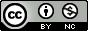Scientific journal NRU ITMO
Series "Refrigeration and Air Conditioning"
Registration certificate ЭЛ № ФС 77 – 55410 от 17.09.2013
зарегистрировано в Федеральной службе по надзору в сфере связи, информационных технологий и массовых коммуникаций
ISSN:2310-1148
Publications

June 2015 (published: 28.05.2015)

Number 2(18)

Home > Issue > The equation of elasticity R236EA

## The equation of elasticity R236EA

The paper proposed the equation of elasticity hexafluoropropane, taking into account the requirements of scale hypotheses and behaviors vapor pressure near the critical and triple points. A comparison with the experimental data on the pressure in the line of elasticity. Revealed good agreement with experiment in the vicinity of the critical point, in contrast to the equations of other authors. Information about the relative deviation is presented in graphical form On the basis of the proposed equation calculated detailed tables, depending on the temperature of the pressure on the line of phase equilibrium. Also in the pre-table shows calculated values of the first and second derivatives of pressure on the elasticity line. The article provides a detailed algorithm for finding the coefficient of elasticity line. The possibility of using the proposed equation in the construction of scale and wide-range equations of state.
Read the full articleKeywords: elastic line, the equation of state, the line of phase equilibrium scaling theory, R236ea, hexafluoropropane.